• +91 9971497814
• info@interviewmaterial.com

# RD Chapter 24- Measures of Central Tendency Ex-24.1 Interview Questions Answers

### Related Subjects

Question 1 : If the heights of 5 persons are 140 cm, 150 cm, 152 cm, 158 cm and 161 cm respectively. Find the mean height.

Heights of 5 persons are 140 cm, 150 cm, 152 cm, 158 cm and 161 cm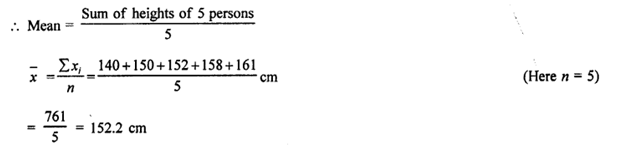Question 2 : Find the mean of 994, 996, 998, 1002 and 1000.

Answer 2 : Mean of 994, 996, 998, 1002 and 1000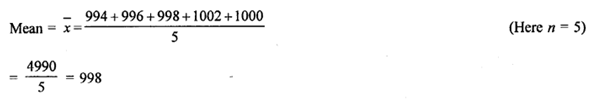Question 3 : Find the mean of first five natural numbers.

First five natural numbers are 1, 2, 3, 4, 5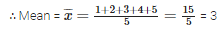Question 4 : Find the mean all factors of 10.

Answer 4 : Factors of 10 = 1, 2, 5, 10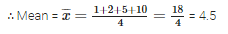Question 5 : Find the mean of first 10 even natural numbers.

Answer 5 : First 10 even natural numbers are 2, 4, 6, 8, 10, 12, 14, 16, 18, 20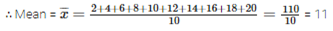Question 6 : Find the mean of x, x + 2, x + 4, x + 6, x + 8.

Answer 6 : Sum = x + x + 2+ x + 4 + x + 6 + x + 8 = 5x + 20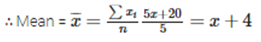Question 7 : Find the mean of first five multiples of 3.

Answer 7 : First 5 multiples of 3 are = 3, 6, 9, 12, 15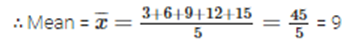Question 8 :
Following are the weights (in kg) or 10 new born babies in a hospital on a particular day:
3.4, 3.6, 4.2, 4.5, 3.9, 4.1, 3.8, 4.5, 4.4, 3.6. Find the mean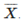Answer 8 : Weights of 10 new bom babies are 3.4, 3.6, 4.2, 4.5, 3.9, 4.1, 3.8, 4.5, 4.4, 3.6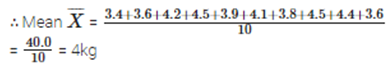Question 9 : The percentage of marks obtained by students of a class in mathematics are : 64, 36, 47, 23, 0, 19, 81, 93, 72, 35, 3, 1. Find their mean.

Answer 9 : Percentage of 12 students are 64, 36, 47, 23, 0, 19, 81, 93, 72, 35, 3, 1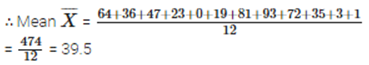Question 10 : The numbers of children in 10 families of a locality are : 2, 4, 3, 4, 2, 0, 3, 5, 1, 1, 5. Find the mean number of children per family.

Answer 10 : Number of children in 10 families are 2, 4, 3, 4, 2, 0, 3, 5, 1, 1, 5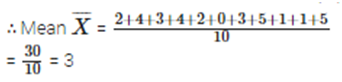Todays Deals### RD Chapter 24- Measures of Central Tendency Ex-24.1 Contributorskrishan

Name:
Email:

# Latest News# 9000 interview questions in different categories